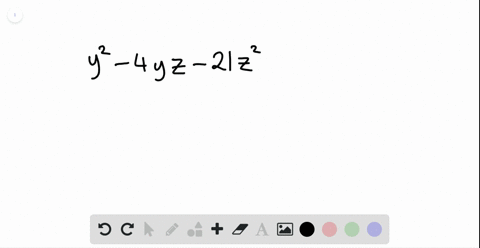Enroll in one of our FREE online STEM summer camps. Space is limited so join now!View Summer Courses### Factor each polynomial. If a polynomial cannot be…

01:09Other Schools

Need more help? Fill out this quick form to get professional live tutoring.

Get live tutoring
Problem 10

Factor each polynomial. If a polynomial cannot be factored, write prime. Factor out the greatest common factor as necessary.
$$s^{2}+2 s t-35 t^{2}$$

$s^{2}+2 s t-35 t^{2}=(s-5 t)(s+7 t)$

## Discussion

You must be signed in to discuss.

## Video Transcript

we're asked to factor this train A meal here. The first term is that skirt. So that means are factors must both start with us. The last term is negative. 35 t squared. So numbers must be something. Multiply tea and another number multiplied by t. And those two numbers here must multiply together to be negative 35 and add together to be positive too. So if we list are factors of negative 35 impairs, we would have negative one comma Positive. 35 native five Positive seven Negative seven Positive five The negative 35 Positive one. So out of these pears on Lee the negative five comma seven Multiply together. Sorry. Ad together to be positive too. So this means are factors Air s minus five T and s plus 70